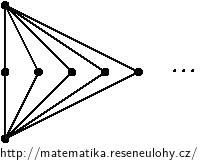## Complete bipartite graphs

Show that the graph $$K_{m, n}$$ is planar if and only if $$\min \{m, n \} \le 2$$.
If $$\min \{m, n \} \ge 3$$, then the graph contains $$K_{3{,}3}$$ as a subgraph and is not planar according to the Kuratowski’s theorem.
The graph $$K_{2, n}$$ can be drawn in a planar way, see the picture. Graphs $$K_{1, n}$$ and $$K_{0, n}$$ are its subgraphs, so they are also planar.